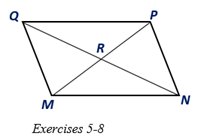Chapter 4.1, Problem 8E### Elementary Geometry for College St...

6th Edition
Daniel C. Alexander + 1 other
ISBN: 9781285195698

#### Solutions

Chapter
Section### Elementary Geometry for College St...

6th Edition
Daniel C. Alexander + 1 other
ISBN: 9781285195698
Textbook Problem
25 views

# For Exercises 5 to 8, MNPQ is a parallelogram with diagonals Q N - and M P - .If M R = 5 ( a + 7 ) and M P = 12 a + 34 , find MR, RP, and MP.

To determine

To find:

The lengths of the MR, RP, and MP in the parallelogram MNPQ.

Explanation

Given:

MNPQ is a parallelogram with diagonals QN- and MP- and MR=5(a+7) and MP=12a+34.

Corollary:

The diagonals of a parallelogram bisect each other.

Calculation:

In the given parallelogram MNPQ, given that the diagonals QN- and MP- and MR=5(a+7) and MP=12a+34.

As per the corollary that the diagonals of a parallelogram bisect each other, the diagonals QN- and MP- bisect each other at R.

So MR=RP=12MP

Substituting MR=5(a+7) and MP=12a+34 in MR=12MP

5(a+7)=12×12a+34

### Still sussing out bartleby?

Check out a sample textbook solution.

See a sample solution

#### The Solution to Your Study Problems

Bartleby provides explanations to thousands of textbook problems written by our experts, many with advanced degrees!

Get Started

#### Simplify: 915

Elementary Technical Mathematics

#### 30. If

Mathematical Applications for the Management, Life, and Social Sciences

#### For what values of p does the series converge?

Study Guide for Stewart's Multivariable Calculus, 8th

#### (y)2 + 6xy 2y + 7x = 0 is a differential equation of order ______. a) 1 b) 2 c) 3 d) 4

Study Guide for Stewart's Single Variable Calculus: Early Transcendentals, 8th

#### Describe the purpose of a dismantling design.

Research Methods for the Behavioral Sciences (MindTap Course List)